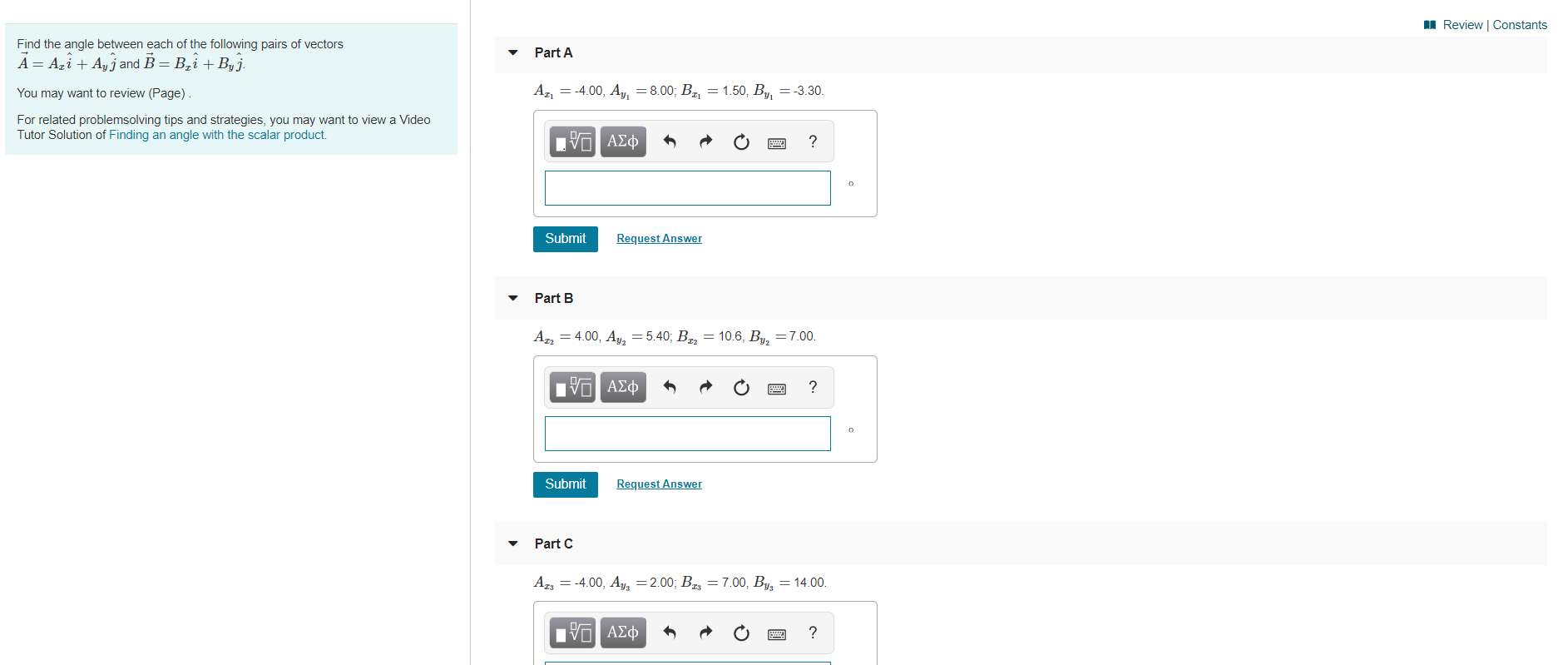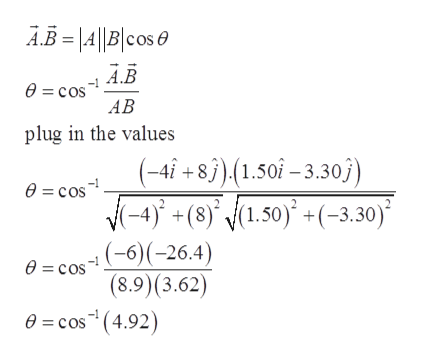# Review | ConstantsFind the angle between each of the following pairs of vectorsPart AA AziAjand B B2i Byj.= -3.30.A24.00, Ay, = 8.00; B2, = 1.50, BYou may want to review (Page)For related problemsolving tips and strategies, you may want to view a VideoTutor Solution of Finding an angle with the scalar product.Eνα ΑΣφRequest AnswerSubmitPart BAT4.00, Ay=5.40; B= 106, By, = 7.00.| ΑΣφ?SubmitRequest AnswerPart CAI34.00, As = 2.00; Bg = 7.00, B = 14.00oΠνΟ ΑΣφE

Question
12 views

Can you solve and show steps? thanks.help_outlineImage TranscriptioncloseReview | Constants Find the angle between each of the following pairs of vectors Part A A AziAjand B B2i Byj. = -3.30. A2 4.00, Ay, = 8.00; B2, = 1.50, B You may want to review (Page) For related problemsolving tips and strategies, you may want to view a Video Tutor Solution of Finding an angle with the scalar product. Eνα ΑΣφ Request Answer Submit Part B AT4.00, Ay=5.40; B = 106, By, = 7.00. | ΑΣφ ? Submit Request Answer Part C AI34.00, As = 2.00; Bg = 7.00, B = 14.00o ΠνΟ ΑΣφ E fullscreen
check_circle

Step 1

Given:

For part (A):

Step 2

Angle between the vectors by scalar product can be calculated as:help_outlineImage TranscriptioncloseAB=|A||B|cos A.B 0= cos AB plug in the values (-4i + 83),(1.50î-3.30j) e = cos (-4)+(8)', (1.50) +(-3.30) (-6)(-26.4) (8.9)(3.62) 0=cos (4.92) e = cos fullscreen
Step 3

Given

part (B...

### Want to see the full answer?

See Solution

#### Want to see this answer and more?

Solutions are written by subject experts who are available 24/7. Questions are typically answered within 1 hour.*

See Solution
*Response times may vary by subject and question.
Tagged in

### Vectors and Scalars